Ex 12.2

Chapter 12 Class 10 Areas related to Circles
Serial order wise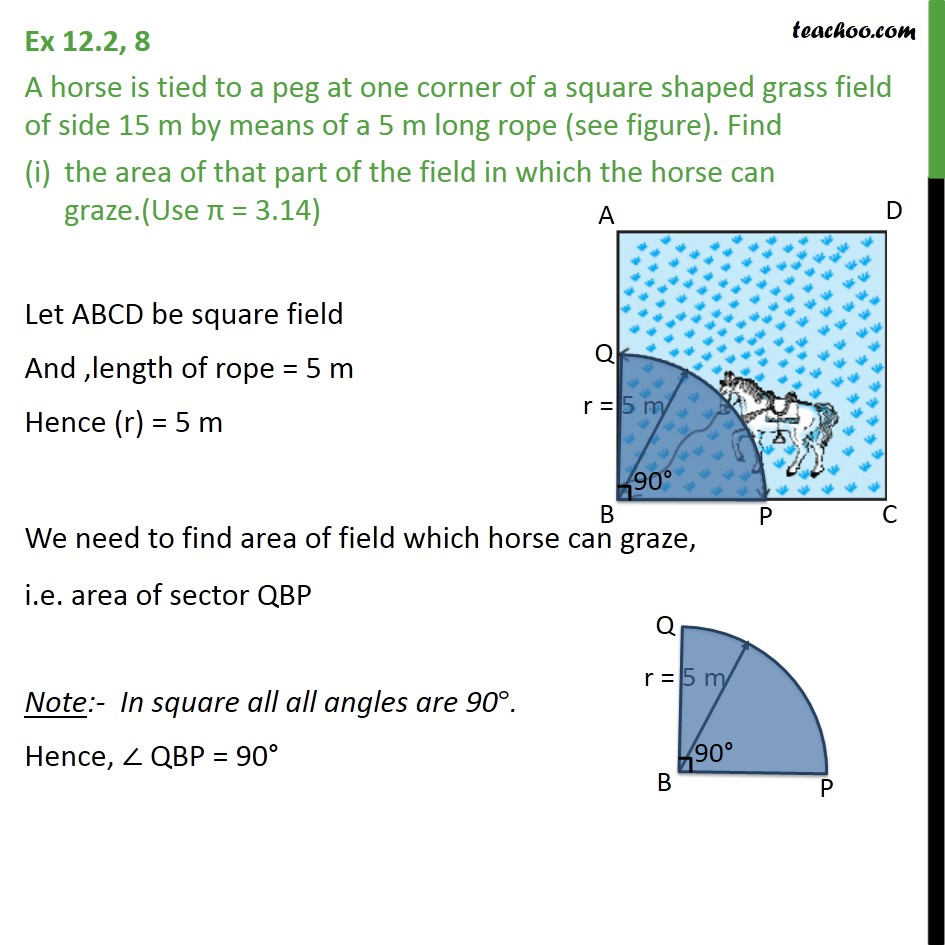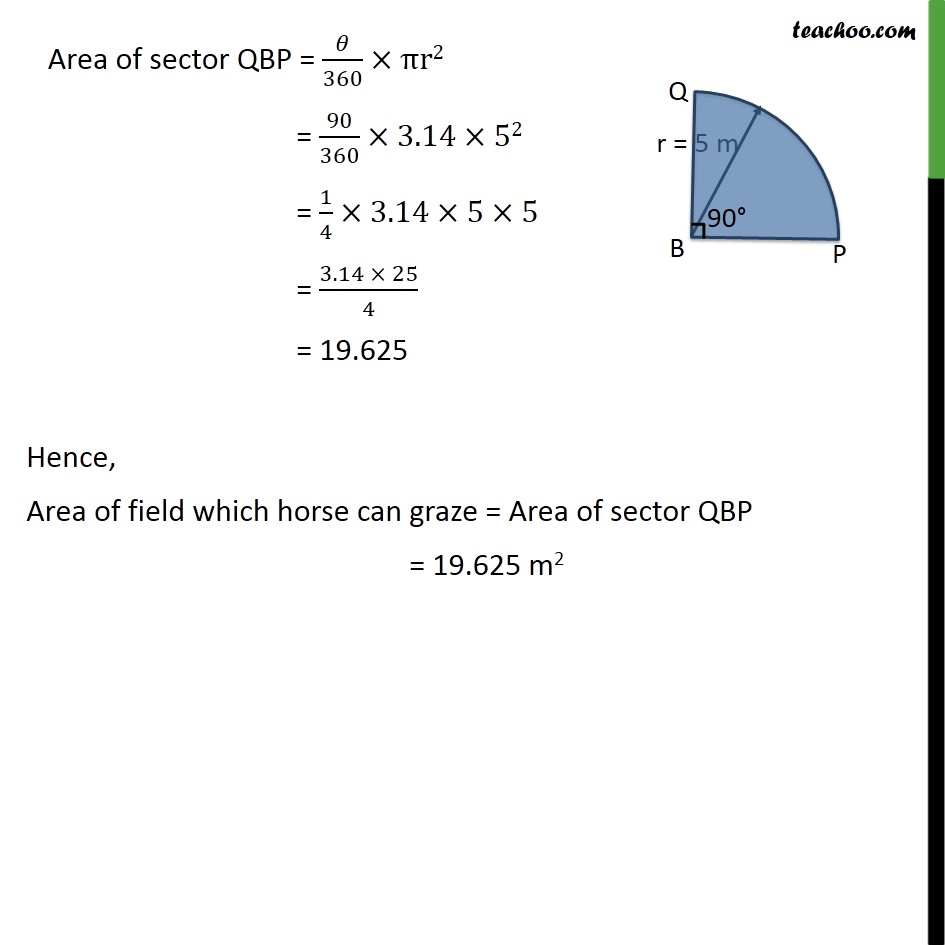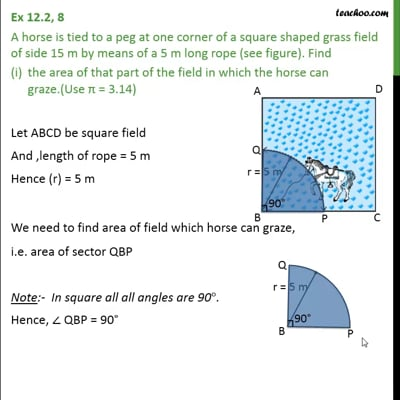This video is only available for Teachoo black users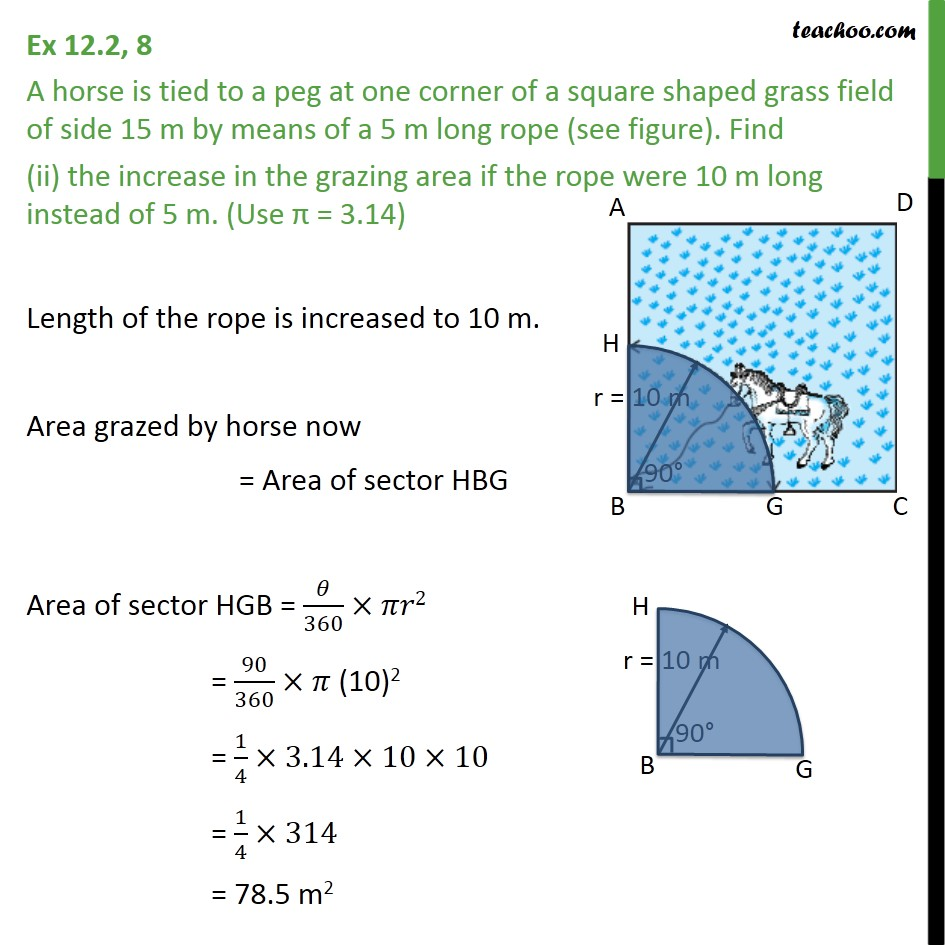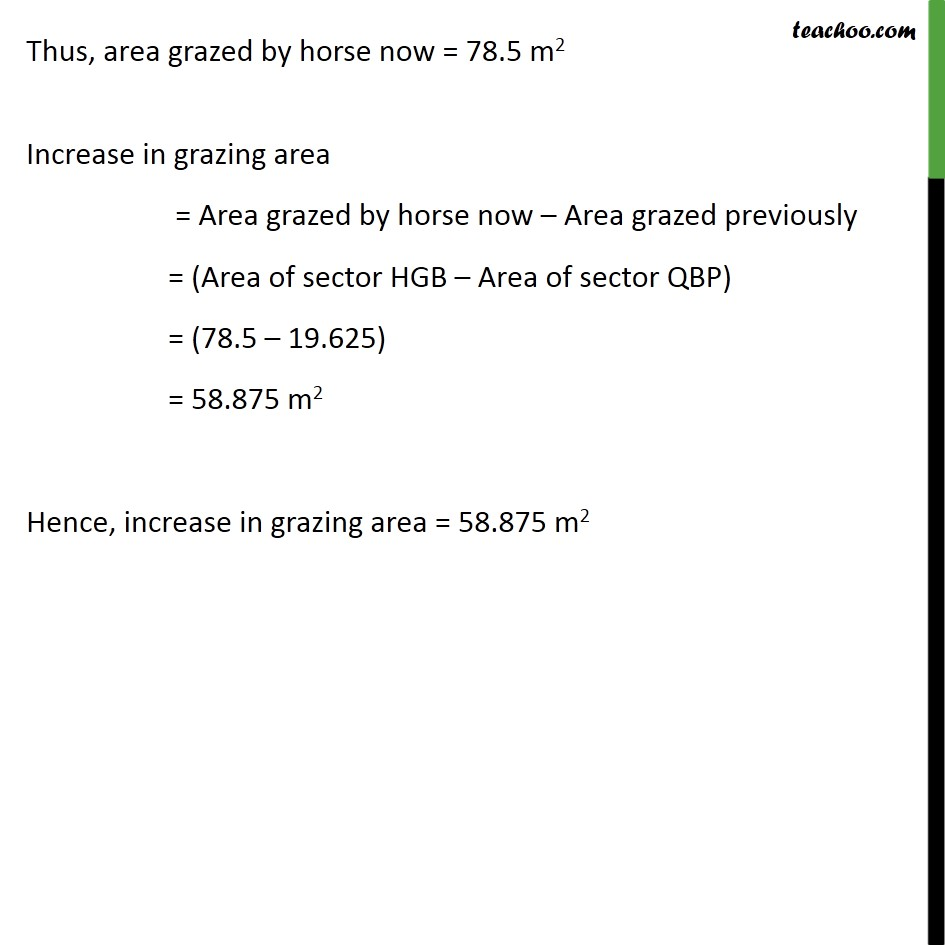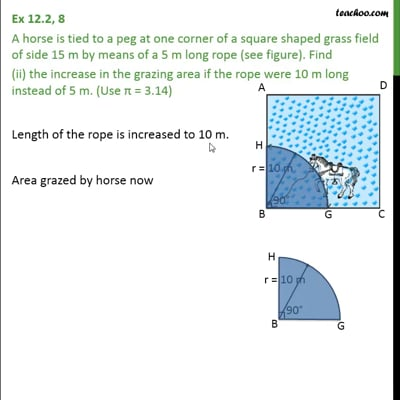This video is only available for Teachoo black users

Solve all your doubts with Teachoo Black (new monthly pack available now!)

### Transcript

Ex 12.2, 8 A horse is tied to a peg at one corner of a square shaped grass field of side 15 m by means of a 5 m long rope (see figure). Find the area of that part of the field in which the horse can graze.(Use π = 3.14) Let ABCD be square field And ,length of rope = 5 m Hence (r) = 5 m We need to find area of field which horse can graze, i.e. area of sector QBP Note:- In square all all angles are 90°. Hence, ∠ QBP = 90° Area of sector QBP = 𝜃/360×πr2 = 90/360×3.14×52 = 1/4×3.14×5×5 = (3.14 × 25)/4 = 19.625 Hence, Area of field which horse can graze = Area of sector QBP = 19.625 m2 Ex 12.2, 8 A horse is tied to a peg at one corner of a square shaped grass field of side 15 m by means of a 5 m long rope (see figure). Find (ii) the increase in the grazing area if the rope were 10 m long instead of 5 m. (Use π = 3.14) Length of the rope is increased to 10 m. Area grazed by horse now = Area of sector HBG Area of sector HGB = 𝜃/360×𝜋𝑟2 = 90/360×𝜋 (10)2 = 1/4×3.14×10×10 = 1/4×314 = 78.5 m2 Thus, area grazed by horse now = 78.5 m2 Increase in grazing area = Area grazed by horse now – Area grazed previously = (Area of sector HGB – Area of sector QBP) = (78.5 – 19.625) = 58.875 m2 Hence, increase in grazing area = 58.875 m2